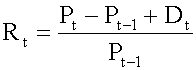Subject 2. Holding Period Return
When analyzing rates of return, our starting point is the total return, or holding period return (HPR). HPR measures the total return for holding an investment over a certain period of time, and can be calculated using the following formula:• Pt = price per share at the end of time period t
• P(t-1) = price per share at the end of time period t-1, the time period immediately preceding time period t
• Pt - Pt-1 = price appreciation of the investment
• Dt = cash distributions received during time period t: for common stock, cash distribution is the dividend; for bonds, cash distribution is the coupon payment.
It has two important characteristics:
• It has an element of time attached to it: monthly, quarterly or annual returns. HPR can be computed for any time period.
• It has no currency unit attached to it; the result holds regardless of the currency in which prices are denominated.
Example

A stock is currently worth $60. If you purchased the stock exactly one year ago for$50 and received a $2 dividend over the course of the year, what is your holding period return? Rt = ($60 - $50 +$2)/\$50 = 0.24 or 24%

The return for time period t is the capital gain (or loss) plus distributions divided by the beginning-of-period price (dividend yield). Note that for common stocks the distribution is the dividend; for bonds, the distribution is the coupon payment.

The holding period return for any asset can be calculated for any time period (day, week, month, or year) simply by changing the interpretation of the time interval.

Return can be expressed in decimals (0.05), fractions (5/100), or as a percent (5%). These are all equivalent.

If you want to change selection, open original toplevel document below and click on "Move attachment"